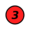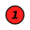www.postcogito.org           ...sine propero notiones

Kiko

 You are here: Kiko > EmbeddedSystems > BcdClock > TheBinaryClockInBCDGrayMode Printable | topic end

# The Binary Clock in BCD Gray Mode

Instructions to read a binary clock in BCD+Gray Code mode, along with a visual JavaScript simulation with hints.

## JavaScript Simulation1 7 4 1 2 3 HOURS MINUTES SECONDS
 Hints: LED Values Decimal

If your browser supports JavaScript and you have it enabled, the example above should be "live", showing the current time from your computer's clock and updated once a second just like the real-life binary clock, but with extra hints to help you understand the display. Click on the checkboxes to disable/reenable the various levels of hits as you gain experience.

If your browser does not support JavaScript or you don't have it enabled, the picture above will be static, sorry.

## How to read the time in BCD Gray Mode

This is the same as the standard BCD mode but with a small difference: each column (=decimal digit) is encoded in Binary-Reflected Gray Code (BRGC).

A Gray Code is one of many ways to count in binary such that from one number to the other we change only one bit. There are several varieties of Gray codes; the most famous is probably the Binary Reflected variety.

As in the clock there are only the ten possible bit patters listed below, it is just as easy as the original BCD mode:0 1 2 3 4 5 6 7 8 9

Notice that from 0 to 1, only one bit changes: LED "1" lights. From 1 to 2, led "3" lights -- again only one LED changes. From 2 to 3, LED "1", that was previously on, goes off. And so on -- from one transition to the other, only one LED changes, either from on to off or the other way around.

If we went all the way to the transition form 15 back to 0, the propery of only one bit changing would be maintained. However, since we truncate the sequence at 9, the transition from 9 to 0 makes three bits change at the same time.

But if you want to calculate instead of memorize the table above, here's how to do it: start from the largest-valued (topmost, in those drawings) LED that is on. Subtract this from the value of the next LED below that is on. Then add the value from the next LED below that is on. Then subtract, then add, then subtract, then add, and so on.

And how to calculate the table? Simple: the bottom row is one. Each row above it is twice the row below it, plus one. So the second row is 2*1+1 = 3; the third is 2*3+1 = 7; the fourh is 2*7+1. In general, the nth row from the bottom up is 2n-1+1.
top

You are here: Kiko > EmbeddedSystems > BcdClock > TheBinaryClockInBCDGrayMode

topThe content of this site is made available under the terms of a Creative Commons License, except where otherwise noted.
O conteúdo deste site está disponibilizado nos termos de uma Licença Creative Commons, exceto onde dito em contrário.